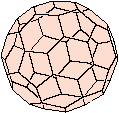Balancing Nuclear EquationsAlpha Emission

The three most common types of radioactive emission are alpha, beta and gamma. For example, when a Uranium-238 nucleus decays, it produces a Thorium-234 nucleus and a Helium-4 nucleus. That Helium-4 nucleus is called an alpha particle(α).

 238 234 4 U → Th + He 92 90 2

There are two important things to note here:
• The sum of the isotopic masses (top numbers) are equal on both sides of the equation. [238 =234 + 4]
• The sum of the atomic numbers (the bottom numbers) also are equal on both sides of the equation. [92 = 90 + 2]